# 四、图像增强

• 逐点强度变换–像素变换
• 直方图处理、直方图均衡化、直方图匹配
• 线性噪声平滑（平均滤波器）
• 非线性噪声平滑（中值滤波器）

# 对数变换

def plot_image(image, title=''):
pylab.title(title, size=20), pylab.imshow(image)
pylab.axis('off') # comment this line if you want axis ticks

def plot_hist(r, g, b, title=''):
r, g, b = img_as_ubyte(r), img_as_ubyte(g), img_as_ubyte(b)
pylab.hist(np.array(r).ravel(), bins=256, range=(0, 256), color='r', alpha=0.5)
pylab.hist(np.array(g).ravel(), bins=256, range=(0, 256), color='g', alpha=0.5)
pylab.hist(np.array(b).ravel(), bins=256, range=(0, 256), color='b', alpha=0.5)
pylab.xlabel('pixel value', size=20), pylab.ylabel('frequency', size=20)
pylab.title(title, size=20)

im = Image.open("../images/parrot.png")
im_r, im_g, im_b = im.split()
pylab.style.use('ggplot')
pylab.figure(figsize=(15,5))
pylab.subplot(121), plot_image(im, 'original image')
pylab.subplot(122), plot_hist(im_r, im_g, im_b,'histogram for RGB channels')
pylab.show()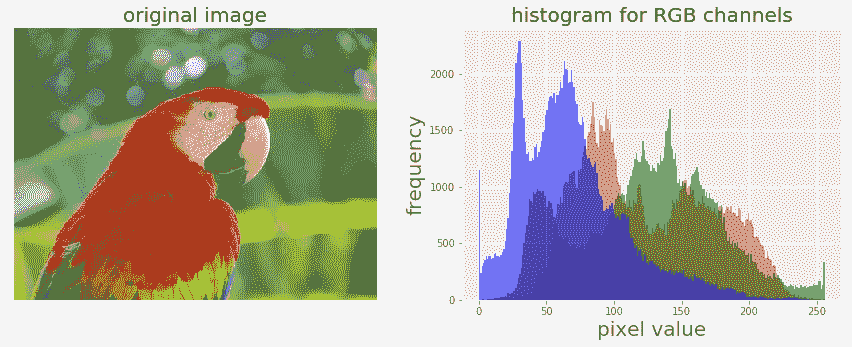im = im.point(lambda i: 255*np.log(1+i/255))
im_r, im_g, im_b = im.split()
pylab.style.use('ggplot')
pylab.figure(figsize=(15,5))
pylab.subplot(121), plot_image(im, 'image after log transform')
pylab.subplot(122), plot_hist(im_r, im_g, im_b, 'histogram of RGB channels log transform')
pylab.show()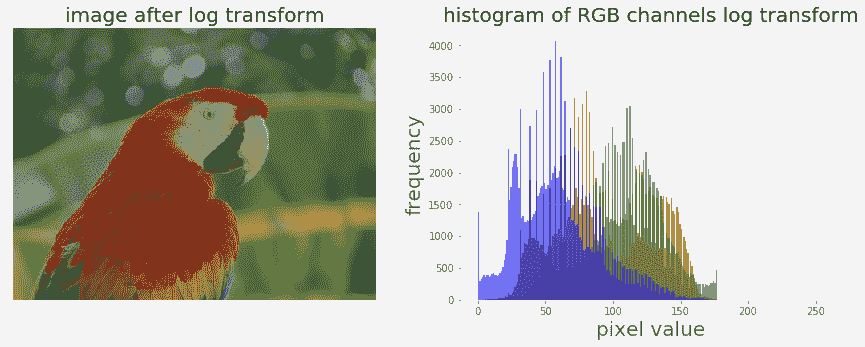# 幂律变换

im = img_as_float(imread('../images/earthfromsky.jpg'))gamma = 5im1 = im**gammapylab.style.use('ggplot')pylab.figure(figsize=(15,5))pylab.subplot(121), plot_hist(im[...,0], im[...,1], im[...,2], 'histogram for RGB channels (input)')pylab.subplot(122), plot_hist(im1[...,0], im1[...,1], im1[...,2], 'histogram for RGB channels ...


# 对比拉伸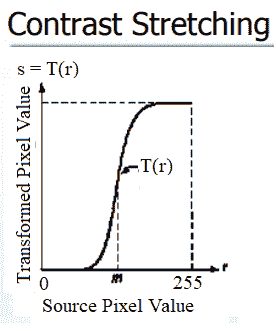# 使用 PIL 作为点操作

im = Image.open('../images/cheetah.png')im_r, im_g, im_b, _ = im.split()pylab.style.use('ggplot')pylab.figure(figsize=(15,5))pylab.subplot(121)plot_image(im)pylab.subplot(122)plot_hist(im_r, im_g, im_b)pylab.show()


# 使用 PIL 图像增强模块

ImageEnhance模块也可用于对比度拉伸。下面的代码块显示了如何使用来自对比度对象的enhance()方法来增强相同输入图像的对比度：

contrast = ImageEnhance.Contrast(im)
im1 = np.reshape(np.array(contrast.enhance(2).getdata()).astype(np.uint8), (im.height, im.width, 4))
pylab.style.use('ggplot')
pylab.figure(figsize=(15,5))
pylab.subplot(121), plot_image(im1)
pylab.subplot(122), plot_hist(im1[...,0], im1[...,1], im1[...,2]), pylab.yscale('log',basey=10)
pylab.show()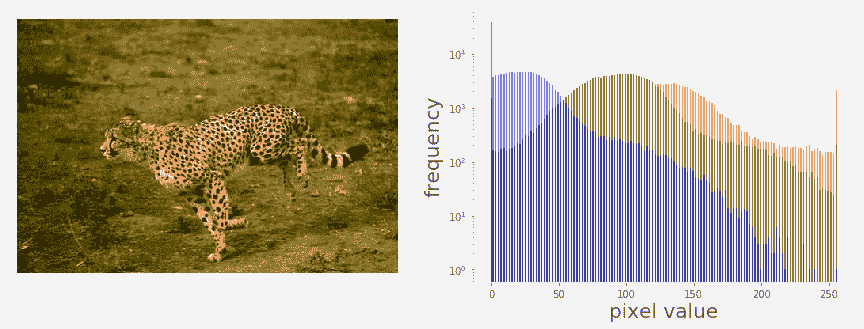# 阈值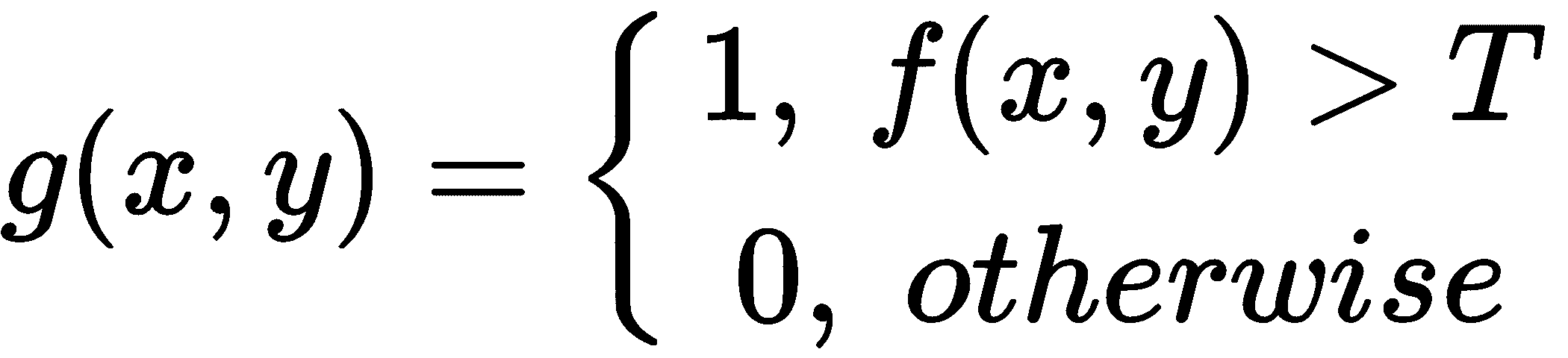# 有固定的门槛

im = Image.open('../images/swans.jpg').convert('L')
pylab.hist(np.array(im).ravel(), bins=256, range=(0, 256), color='g')
pylab.xlabel('Pixel values'), pylab.ylabel('Frequency'),
pylab.title('Histogram of pixel values')
pylab.show()
pylab.figure(figsize=(12,18))
pylab.gray()
pylab.subplot(221), plot_image(im, 'original image'), pylab.axis('off')
th = [0, 50, 100, 150, 200]
for i in range(2, 5):
im1 = im.point(lambda x: x > th[i])
pylab.subplot(2,2,i), plot_image(im1, 'binary image with threshold=' + str(th[i]))
pylab.show()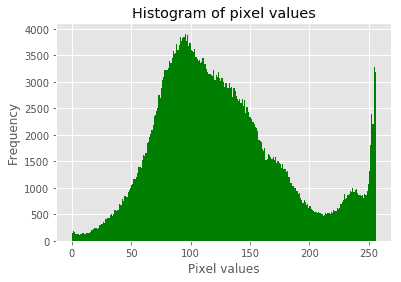*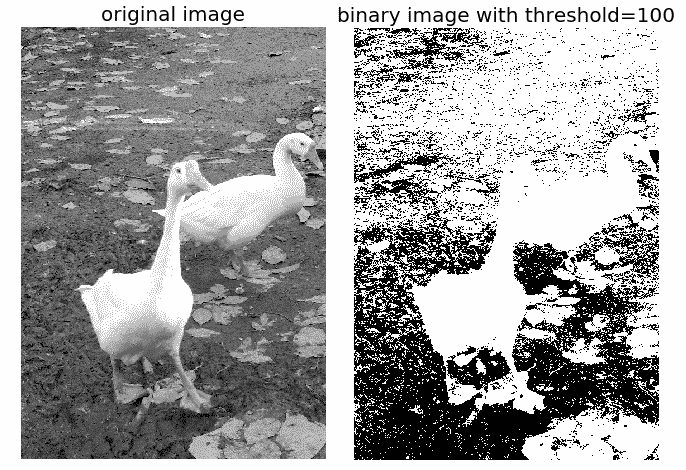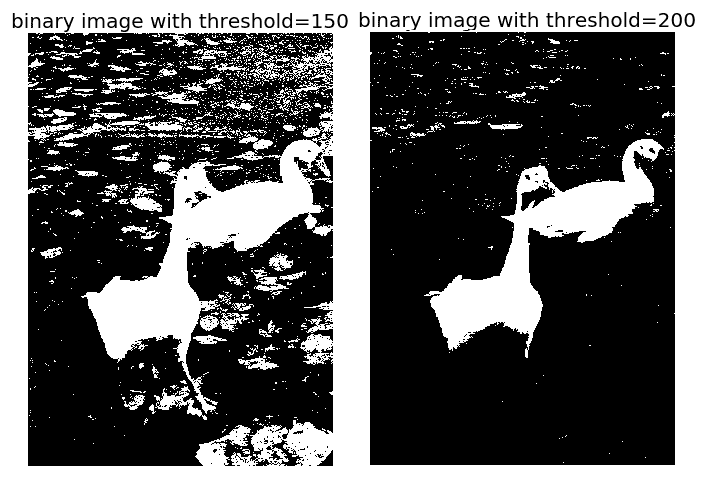# 半色调

im = Image.open('../images/swans.jpg').convert('L')im = Image.fromarray(np.clip(im + np.random.randint(-128, 128, (im.height, im.width)), 0, 255).astype(np.uint8))pylab.figure(figsize=(12,18))pylab.subplot(221), plot_image(im, 'original image (with noise)')th = [0, 50, 100, 150, 200]for i in range(2, 5): im1 = im.point(lambda ...


# 具有误差扩散的 Floyd-Steinberg 抖动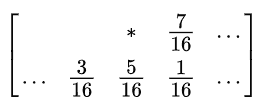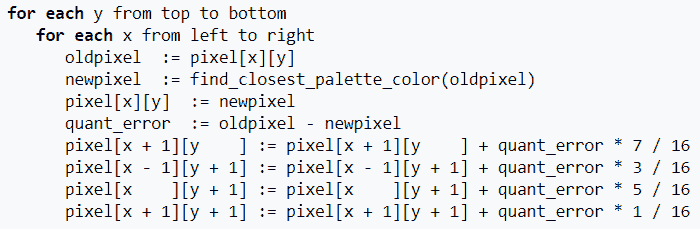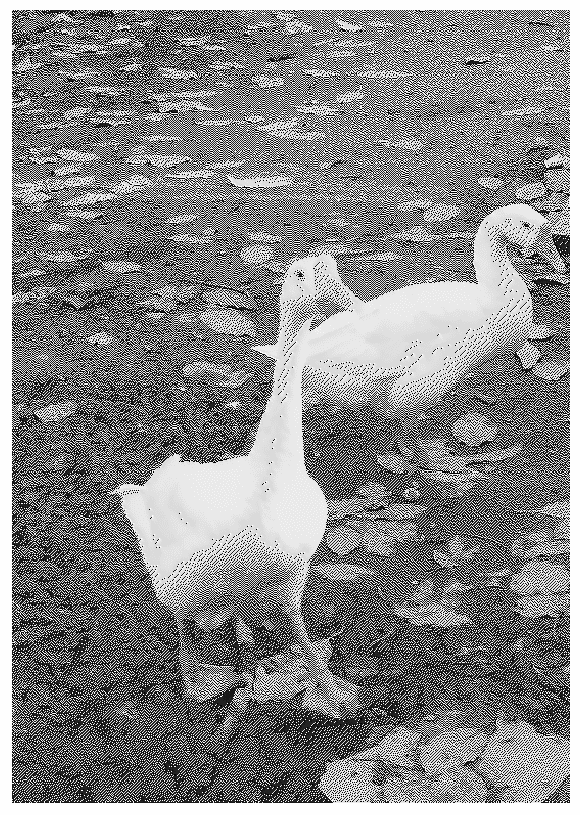# 基于 scikit 图像的对比度拉伸和直方图均衡化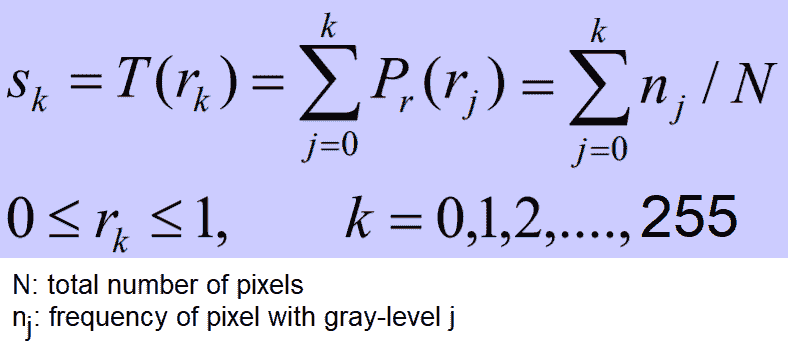img = rgb2gray(imread('../images/earthfromsky.jpg'))
# histogram equalization
img_eq = exposure.equalize_hist(img)
pylab.gray()
titles = ['original input (earth from sky)', 'after histogram equalization', 'after adaptive histogram equalization']
for i in range(3):
pylab.figure(figsize=(20,10)), plot_image(images[i], titles[i])
pylab.figure(figsize=(15,5))
for i in range(3):
pylab.subplot(1,3,i+1), pylab.hist(images[i].ravel(), color='g'), pylab.title(titles[i], size=15)
pylab.show()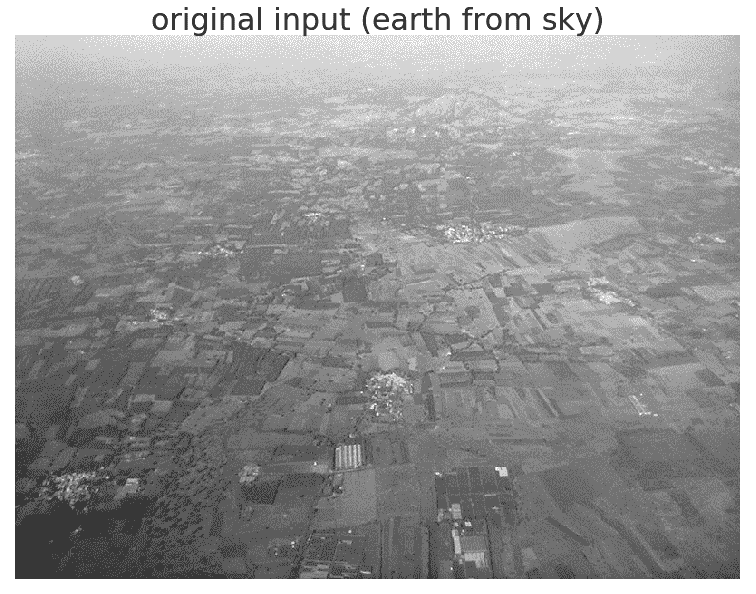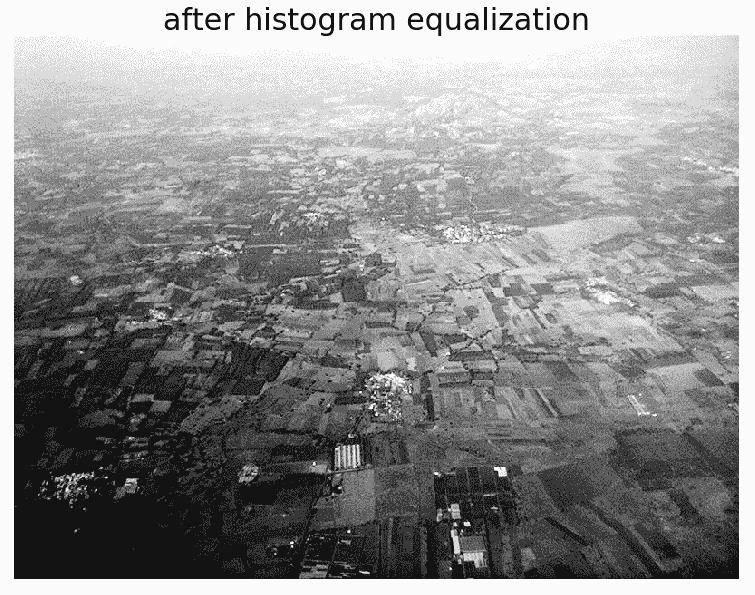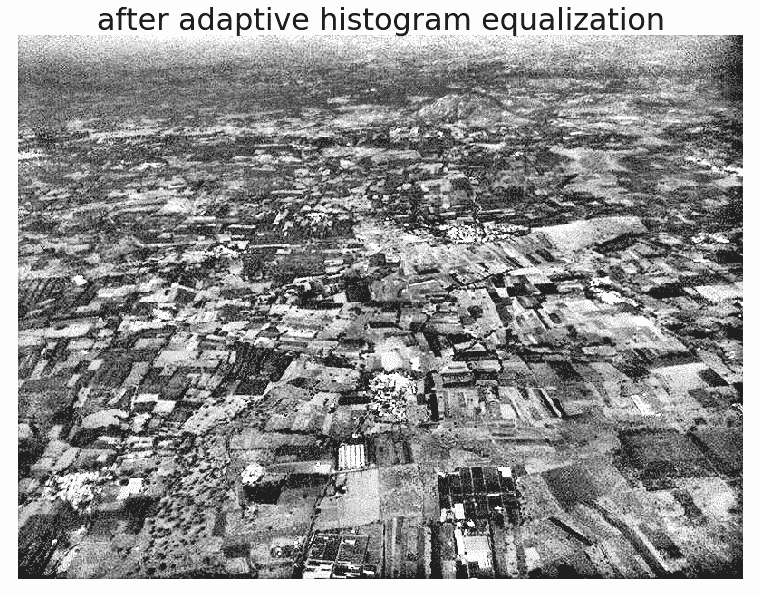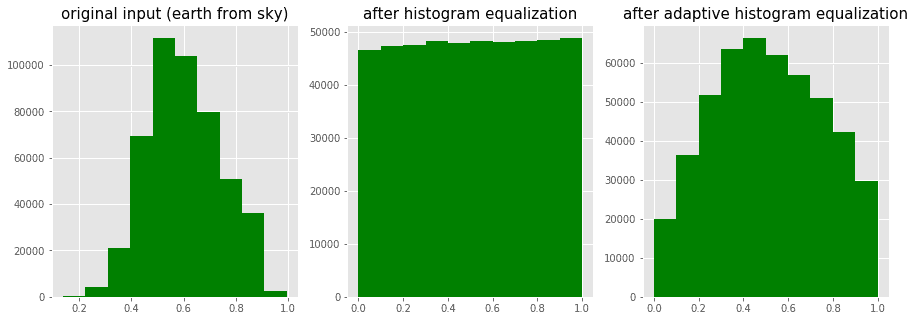import matplotlib
matplotlib.rcParams['font.size'] = 8
def plot_image_and_hist(image, axes, bins=256):
image = img_as_float(image)
axes_image, axes_hist = axes
axes_cdf = axes_hist.twinx()
axes_image.imshow(image, cmap=pylab.cm.gray)
axes_image.set_axis_off()
axes_hist.hist(image.ravel(), bins=bins, histtype='step', color='black')
axes_hist.set_xlim(0, 1)
axes_hist.set_xlabel('Pixel intensity', size=15)
axes_hist.ticklabel_format(axis='y', style='scientific', scilimits=(0, 0))
axes_hist.set_yticks([])
image_cdf, bins = exposure.cumulative_distribution(image, bins)
axes_cdf.plot(bins, image_cdf, 'r')
axes_cdf.set_yticks([])
return axes_image, axes_hist, axes_cdf

# contrast stretching
im_rescale = exposure.rescale_intensity(im, in_range=(0, 100), out_range=(0, 255))
im_eq = exposure.equalize_hist(im) # histogram equalization

fig = pylab.figure(figsize=(15, 7))
axes = np.zeros((2, 4), dtype = np.object)
axes[0, 0] = fig.add_subplot(2, 4, 1)
for i in range(1, 4):
axes[0, i] = fig.add_subplot(2, 4, 1+i, sharex=axes[0,0], sharey=axes[0,0])
for i in range(0, 4):
axes[1, i] = fig.add_subplot(2, 4, 5+i)
axes_image, axes_hist, axes_cdf = plot_image_and_hist(im, axes[:, 0])
axes_image.set_title('Low contrast image', size=20)
y_min, y_max = axes_hist.get_ylim()
axes_hist.set_ylabel('Number of pixels', size=20)
axes_hist.set_yticks(np.linspace(0, y_max, 5))
axes_image, axes_hist, axes_cdf = plot_image_and_hist(im_rescale, axes[:,1])
axes_image.set_title('Contrast stretching', size=20)
axes_image, axes_hist, axes_cdf = plot_image_and_hist(im_eq, axes[:, 2])
axes_image.set_title('Histogram equalization', size=20)
axes_image, axes_hist, axes_cdf = plot_image_and_hist(im_adapteq, axes[:,3])
axes_cdf.set_ylabel('Fraction of total intensity', size=20)
axes_cdf.set_yticks(np.linspace(0, 1, 5))
fig.tight_layout()
pylab.show()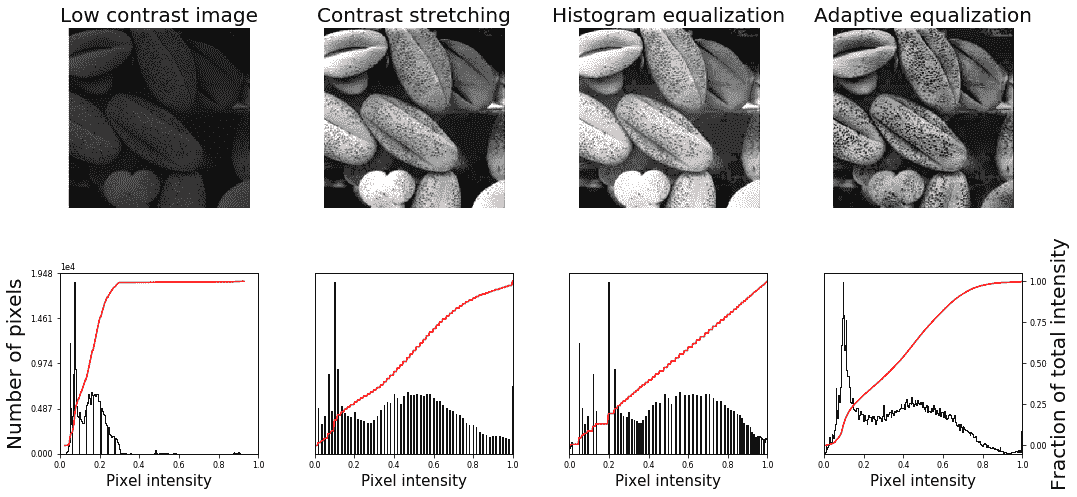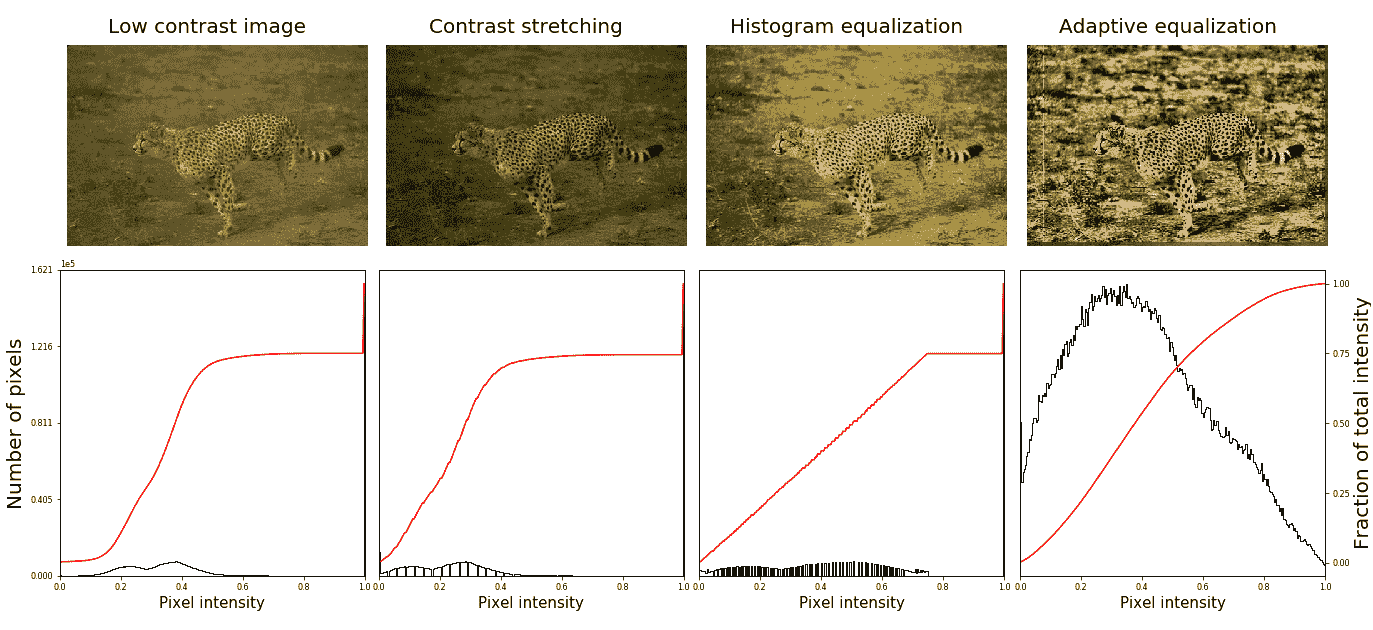# 直方图匹配

1. 将为每个图像计算累积直方图，如以下屏幕截图所示。
2. 对于任意给定的像素值xi【待调整】在输入图像中，我们需要通过匹配输入图像的直方图和模板图像的直方图，在输出图像中找到对应的像素值xj
3. xi像素值具有由G（xi给出的累积直方图值。找到一个像素值xj，使得参考图像中的累积分布值，即H（xj等于【T28 G（xi ** 将输入数据值*xi替换为xj

*# RGB 图像的直方图匹配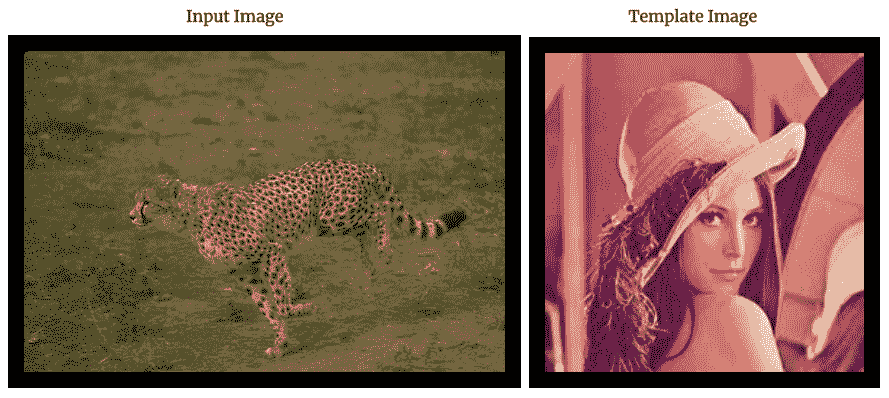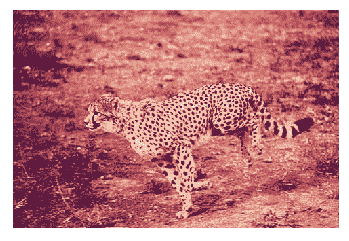# 使用 ImageFilter.BLUR 进行平滑

i = 1pylab.figure(figsize=(10,25))for prop_noise in np.linspace(0.05,0.3,3):    im = Image.open('../images/mandrill.jpg') # choose 5000 random locations inside ...


# 使用框模糊核进行平均平滑

im = Image.open('../images/mandrill_spnoise_0.1.jpg')
pylab.figure(figsize=(20,7))
pylab.subplot(1,3,1), pylab.imshow(im), pylab.title('Original Image', size=30), pylab.axis('off')
for n in [3,5]:
box_blur_kernel = np.reshape(np.ones(n*n),(n,n)) / (n*n)
im1 = im.filter(ImageFilter.Kernel((n,n), box_blur_kernel.flatten()))
pylab.subplot(1,3,(2 if n==3 else 3))
plot_image(im1, 'Blurred with kernel size = ' + str(n) + 'x' + str(n))
pylab.suptitle('PIL Mean Filter (Box Blur) with different Kernel size',
size=30)
pylab.show()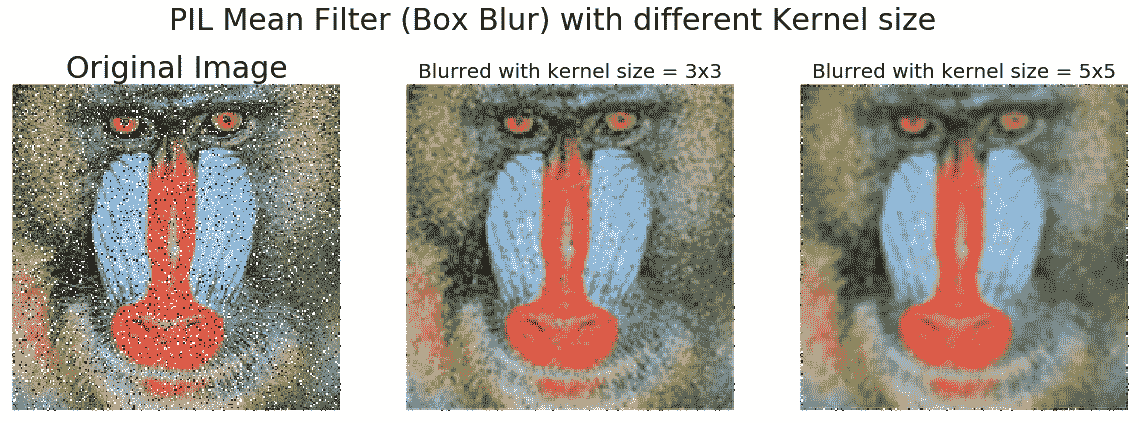# 高斯模糊滤波器的平滑

im = Image.open('../images/mandrill_spnoise_0.2.jpg')pylab.figure(figsize=(20,6))i = 1for radius in range(1, 4):    im1 = im.filter(ImageFilter.GaussianBlur(radius))    pylab.subplot(1,3,i), plot_image(im1, 'radius = ' +    str(round(radius,2)))    i += 1pylab.suptitle('PIL ...


# 使用 SciPy ndimage 比较盒核和高斯核的平滑

from scipy import misc, ndimage
import matplotlib.pylab as pylab
k = 7 # 7x7 kernel
im_box = ndimage.uniform_filter(im, size=(k,k,1))
s = 2 # sigma value
t = (((k - 1)/2)-0.5)/s # truncate parameter value for a kxk gaussian kernel with sigma s
im_gaussian = ndimage.gaussian_filter(im, sigma=(s,s,0), truncate=t)
fig = pylab.figure(figsize=(30,10))
pylab.subplot(131), plot_image(im, 'original image')
pylab.subplot(132), plot_image(im_box, 'with the box filter')
pylab.subplot(133), plot_image(im_gaussian, 'with the gaussian filter')
pylab.show()# PIL 光整

PILImageFilter模块提供一组用于图像非线性去噪的功能。在本节中，我们将用示例演示其中的一些。

# 使用中值滤波器

i = 1pylab.figure(figsize=(25,35))for prop_noise in np.linspace(0.05,0.3,3): ...


# 使用最大和最小过滤器

im = Image.open('../images/mandrill_spnoise_0.1.jpg')
pylab.subplot(1,3,1)
plot_image(im, 'Original Image with 10% added noise')
im1 = im.filter(ImageFilter.MaxFilter(size=sz))
pylab.subplot(1,3,2), plot_image(im1, 'Output (Max Filter size=' + str(sz) + ')')
im1 = im1.filter(ImageFilter.MinFilter(size=sz))
pylab.subplot(1,3,3), plot_image(im1, 'Output (Min Filter size=' + str(sz) + ')', size=15)
pylab.show()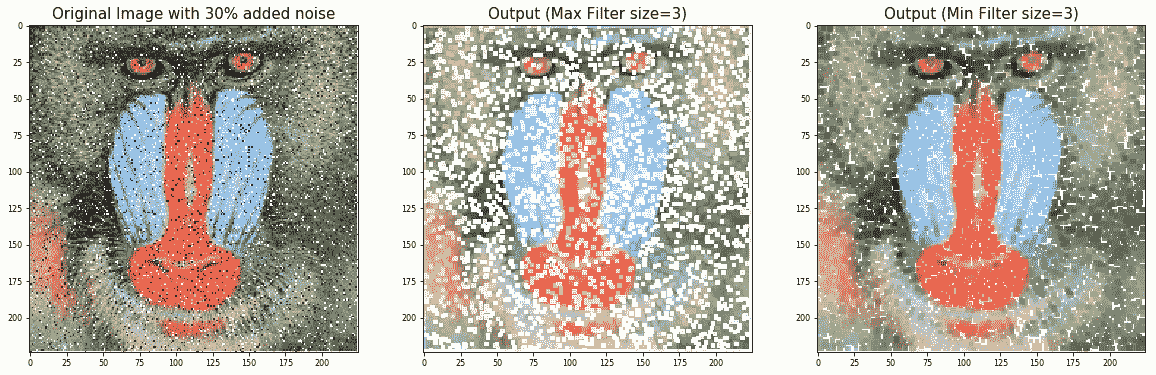# 使用 scikit 图像平滑（去噪）

scikit-image库还在恢复模块中提供了一组非线性滤波器。在以下部分中，我们将讨论两个非常有用的过滤器，即双边和非局部均值过滤器。

# 使用双边滤波器im = color.rgb2gray(img_as_float(io.imread('../images/mountain.png')))
sigma = 0.155
noisy = random_noise(im, var=sigma**2)
pylab.imshow(noisy)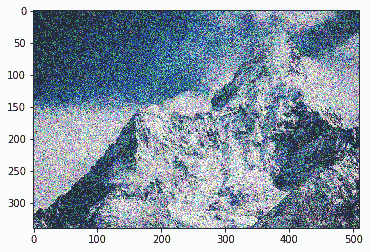pylab.figure(figsize=(20,15))
i = 1
for sigma_sp in [5, 10, 20]:
for sigma_col in [0.1, 0.25, 5]:
pylab.subplot(3,3,i)
pylab.imshow(denoise_bilateral(noisy, sigma_color=sigma_col,
sigma_spatial=sigma_sp, multichannel=False))
pylab.title(r'$\sigma_r=$' + str(sigma_col) + r', $\sigma_s=$' + str(sigma_sp), size=20)
i += 1
pylab.show()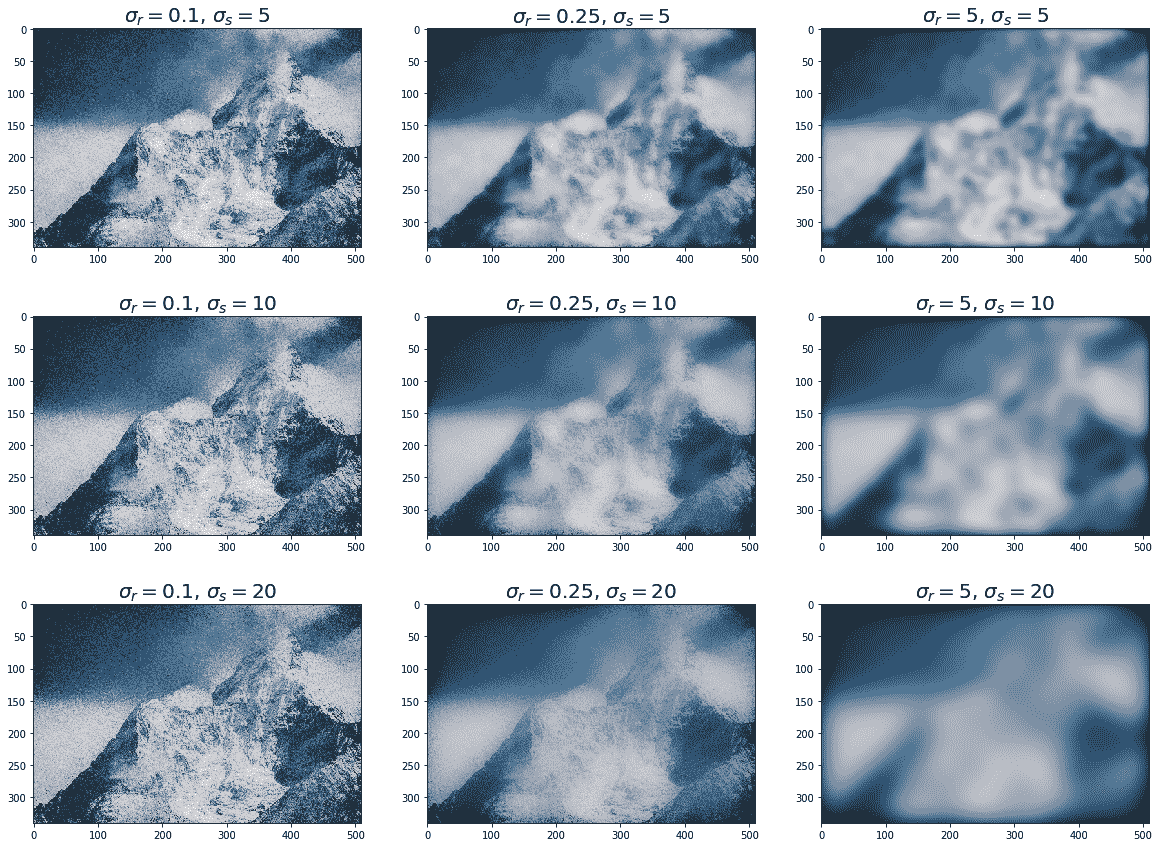# 使用 scipy ndimage 进行平滑

scipyndimage模块提供一个名为percentile_filter()的函数，它是中值滤波器的通用版本。以下代码块演示如何使用此筛选器：

lena = misc.imread('../images/lena.jpg')
# add salt-and-pepper noise to the input image
noise = np.random.random(lena.shape)
lena[noise > 0.9] = 255
lena[noise < 0.1] = 0
plot_image(lena, 'noisy image')
pylab.show()
fig = pylab.figure(figsize=(20,15))
i = 1
for p in range(25, 100, 25):
for k in range(5, 25, 5):
pylab.subplot(3,4,i)
filtered = ndimage.percentile_filter(lena, percentile=p, size=(k,k,1))
plot_image(filtered, str(p) + ' percentile, ' + str(k) + 'x' + str(k) + ' kernel')
i += 1
pylab.show()# 问题

1. 实现彩色 RGB 图像的直方图匹配。
2. 使用skimage.filters.rank中的equalize()函数实现局部直方图均衡化，并将其与具有灰度图像的skimage.exposure中的全局直方图均衡化进行比较。
3. 使用此处描述的算法实现 Floyd Steinberg 误差扩散抖动 https://en.wikipedia.org/wiki/Floyd%E2%80%93Steinberg_dithering 并将灰度图像转换为二值图像。
4. 使用 PIL 中的ModeFilter()对图像进行线性平滑。什么时候有用？
5. 显示一幅图像，该图像可以从几个噪声图像中恢复，这些图像是通过简单地取噪声图像的平均值，将随机高斯噪声添加到原始图像中获得的。中位数也有用吗？